# Quasi Resonant Flyback Design Tool – Automated and Hassle Free

## 1. Setting the Reflected Voltage

Setting the reflected voltage is the first step on this design tool. All the green fields are inputs while yellow fields are outputs.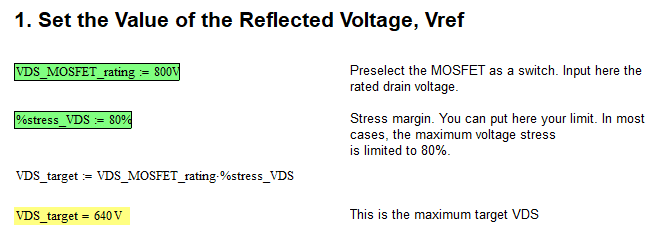## 2. Compute the Input Power

You need to compute the input power using known values. Green fields are inputs, provide data there while yellow fields are calculation results that you should not alter.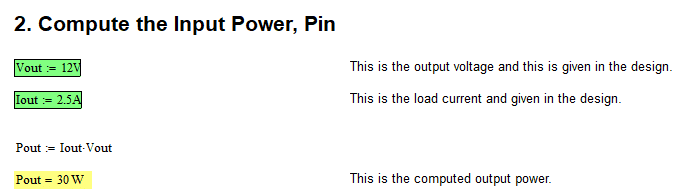## 3. Design the Primary Inductance

In order to ensure getting the full benefit of a quasi resonant flyback converter, the primary inductance must be selected correctly. There is a derivation on how the equation derived but you will not need that anymore as this Quasi resonant flyback design tool will do that for you. All fields in green are inputs while in yellow are computation results.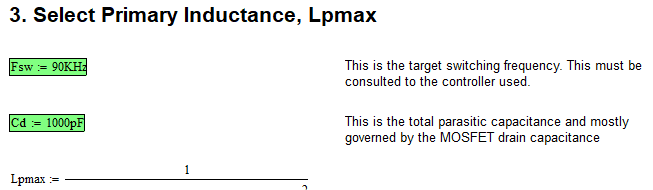## 4. Select the Type of Core

To get going using this QR flyback design tool, you need to select the core material in this step. Supply data on the green fields.## 5. Minimum Number of Turns to the Primary

Insufficient number of turns on the primary winding will make the core saturate. This step will give you the required minimum turns. Fields in green are inputs. Do not alter fields in yellow as they are computation results.## 6. Determine the Turns Ratio

This section will help you determine the primary to secondary turns ratio. Yellow field is a computation result. Do not alter it.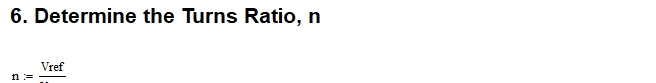## 7. Setting the Secondary Winding Turns

Now determine what turns you need on the secondary winding. Green field is input while yellow field is computation result.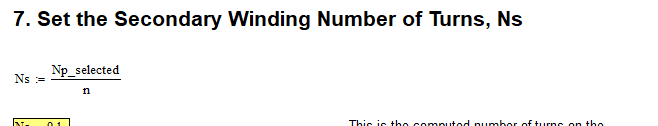Quasi resonant flyback needs dead time to operate as expected. This step will do the calculation. Yellow field is a calculation result.## 9. Determine the Duty Cycle

This step will calculate the duty cycle. Yellow field is a computation result. Don’t alter it.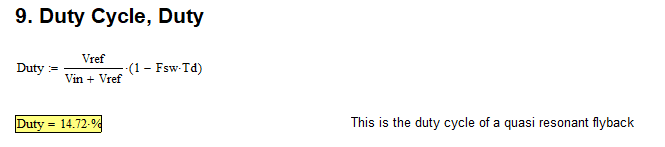## 10. Primary Current Derivation

In this step you will see the values of the primary currents. All fields in yellow are computation results. Do not alter them.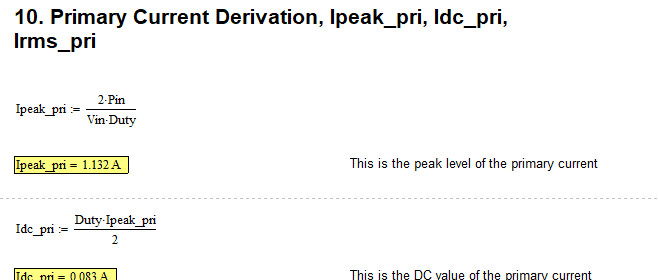## 11. Primary Switch Current Stress

This step will give you tip about the current stress of the switch.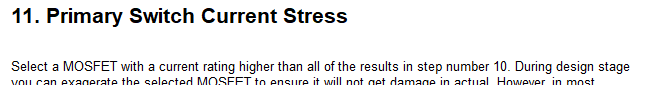## 12. Primary Switch Power Losses

In this step, the power losses of the primary switch will be determined. Green fields are inputs while yellow fields are computation outputs. Do no alter the yellow fields.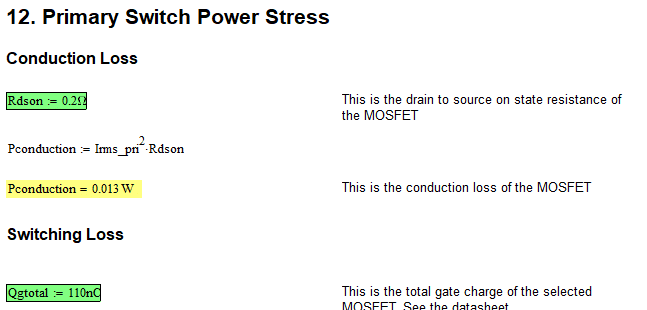## 13. Secondary Current Derivations

You are done with the primary side, its time to go to the secondary. All yellow fields are computation results. Do not alter them.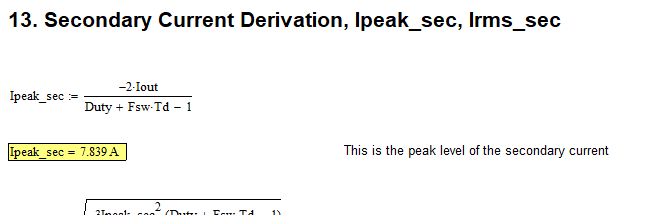## 14. Output Diode Current Stress

This step will give you tip on what should be the stress level be.## 15. Output Bridge Diode Power Stress

This step will give you the power dissipation of the output diode and help you select which part to use. Green fields are input while yellow fields are output.## 16. Output Diode Peak Reverse Voltage

This step will tell you the expected level of the peak reverse voltage. Yellow fields are computation results.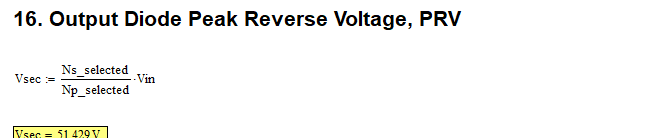## 17. Ripple Current

This step will give the ripple current level. Again, yellow field is a computation result.## 18. Minimum Capacitance

This step will help to compute the minimum needed capacitance on the output. Yellow fields are computation results. Green field is input.## 19. Output Capacitor Selection

Finally, the last step of this quasi resonant flyback design tool is the selection of the output capacitor to ensure a good regulation. Yellow field is computation result.